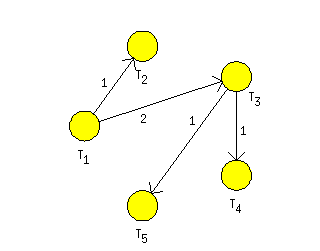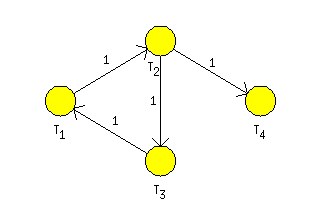"; } ?> Graph Algorithms

# TORSCHE Scheduling Toolbox for Matlab

## TORSCHE – Graph Algorithms

Scheduling algorithms have very close relation to graph algorithms. Scheduling toolbox offer an object graph (see section Chapter 6, Graphs) with several graph algorithms.

### 1. List of Algorithms

List of algorithms related to operations with object graph are summarized in Table 9.1, “List of algorithms”.

algorithmcommandnote
Minimum spanning tree`spanningtree`Polynomial
Dijkstra's algorithm`dijkstra`Polynomial
Floyd`floyd`Polynomial
Minimum Cost Flow`mincostflow`Using LP
Critical Circuit Ratio`criticalcircuitratio`Using LP
Hamilton circuit`hamiltoncircuit`NP-hard
Quadratic Assignment Problem`qap`NP-hard

Table 9.1. List of algorithms

### 2. Minimum Spanning Tree

Spanning tree of the graph is a subgraph which is a tree and connects all the vertices together. A minimum spanning tree is then a spanning tree with minimal sum of the edges cost. A greedy algorithm with polynomial complexity is used to solve this problem (for more details see Demel02). The toolbox function has following syntax:

`gmin = spanningtree(g)`
gmin

minimum spanning tree represented by graph

g

input graph

```>> A = [inf   1  2  inf  7;...
inf inf  3   4  inf;...
inf  9  inf  1   1;...
8   5  inf inf inf;...
7  inf  4   5  inf];
>> g = graph(A);
>> gmin = spanningtree(g);
>> graphedit(gmin);```

Figure 9.1. Spanning tree exampleFigure 9.2. Example of minimum spanning tree

### 3. Dijkstra's Algorithm

Dijkstra's algorithm is an algorithm that solves the single-source cost of shortest path for a directed graph with nonnegative edge weights. Inptut of this algorithm is a directed graph with costs of invidual edges and reference node r from which we want to find shortest path to other nodes. Output is an array with distances to other nodes.

`distances = dijkstra(g,r)`
g

graph object

r

reference node

```>> A = [inf   1  2  inf  7;...
inf inf  3   4  inf;...
inf  9  inf  1   1;...
8   5  inf inf inf;...
7  inf  4   5  inf];
>> g = graph(A);
>> distances = dijkstra(g,2)

distances =

11     0     3     4     4```

Figure 9.3. Dijkstra's algorithm example

### 4. Floyd's Algorithm

Floyd is a well known algorithm from the graph theory [Diestel00]. This algorithm finds a matrix of shortest paths for a given graph. Input to the algorithm is an object graph, where the weights of edges are set in `UserParam` variables of edges. Output is a matrix of shortest paths (U) and optionally matrix of the vertex predecessors (P) in the shortest path and adjacency matrix of lengths (M). Algorithm can be run as follows:

`[U,P,M] = floyd(g)`

The variable `g` is an instance of graph object.

### 5. Strongly Connected Components

The Strongly Connected Components (SCC) of a directed graph are maximal subgraphs for which hold every couple of nodes `u` and `v` there is a path from `u` to `v` and a path from `v` to `u`. For SCC searching Tarjan algorithm is usullay used.

The algorithm is based on depth-first search where the nodes are placed on a stack in the order in which they are visited. When the search returns from a subtree, it is determined whether each node is the root of a SCC. If a node is the root of a SCC, then it and all of the nodes taken off before it form that SCC. The detailed describtion of the algorithm is in [DSVF06]. SCC in a graph G can be found as follows:

`scc = tarjan(g)`

where `scc` is a vector where the element `scc(i)` is number of component where the node `i` belongs to. For graph in Figure 9.5, “A simple network with optimal flow in the fourth user parameter on edges” the algorithm returns fllowing results.

```>> tarjan(g)
ans =
2     2     2     1```

Figure 9.4. Strongly Connected Components example.Figure 9.5. A simple network with optimal flow in the fourth user parameter on edges

### 6. Minimum Cost Flows

The minimum cost flow model is the most fundamental of all network flow problems. In this problem we wish to determine a least cost shipment of a commodity through a network in order to satisfy demands at certain nodes from available supplies at other nodes [Ahuja93]. Let `G=(N,A)` be a directed network defined by set `N` of n nodes and a set `A` of m directed edges. Each edge `(i,j)`